Electricity and Magnetism

# Ampere's law (Quantitative)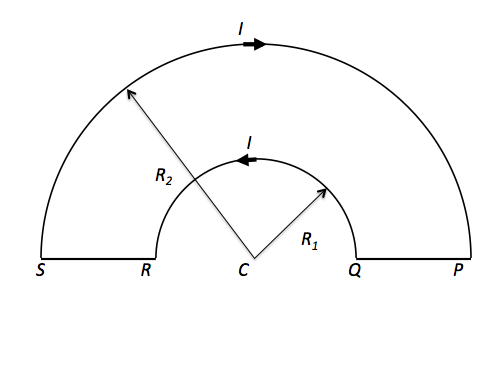The wire loop $PQRSP$ formed by joining two semicircular wires of radius $R_1 = 2 \text{ cm}$ and $R_2 = 4 \text{ cm}$ carries a current of $I = 32 \text{ mA},$ as shown in the figure above. Find the magnitude of the magnetic field at the center $C.$

The magnetic constant is $\mu_0 = 4 \pi \times 10^{-7} \text{ H/m}.$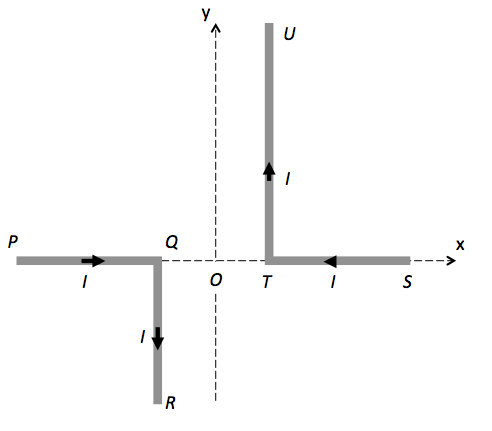The above figure shows two stationary and infinitely long bent wires $PQR$ and $STU$ lying on the $xy$-plane, each carrying a current of $I= 40 \text{ mA}.$ Find the magnitude of the magnetic filed at the origin $O.$

Assume $OQ = OT = a = 2 \text{ cm}.$
The magnetic constant is $\mu_0 = 4 \pi \times 10^{-7} \text{ H/m}.$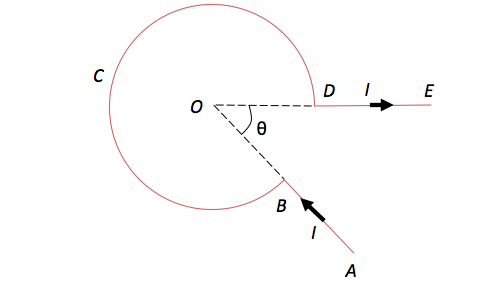A red wire $ABCDE$ is bent, as shown in the figure above. The wire carries a current of $I = 160 \text{ mA},$ and the radius of the bent coil $BCD$ is $r = 5 \text{ cm}.$ If the angle $\angle BOD$ is $\theta = \frac{\pi}{4}$, what is the magnitude of the magnetic field at the center $O?$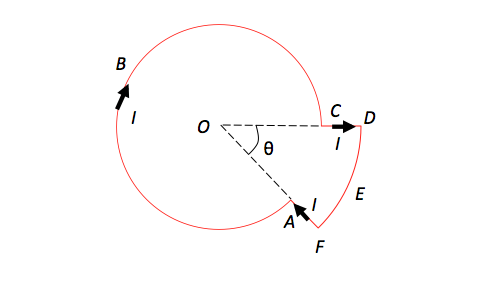A red wire $ABCDEF$ is bent, as shown in the figure above, carrying a current of $I = 54 \text{ mA}.$ The radius of the smaller arc $ABC$ is $r_1 = r = 3 \text{ cm}$ and that of the bigger arc is $r_2 = 2r.$ If the angle $\theta$ in the figure is given by $\theta = 60^\circ = \frac{ \pi}{3} ,$ what is the magnitude of the magnetic field at the center $O?$

The magnetic constant is $mu_0 = 4 \pi \times 10^{-7} \text{ H/m}.$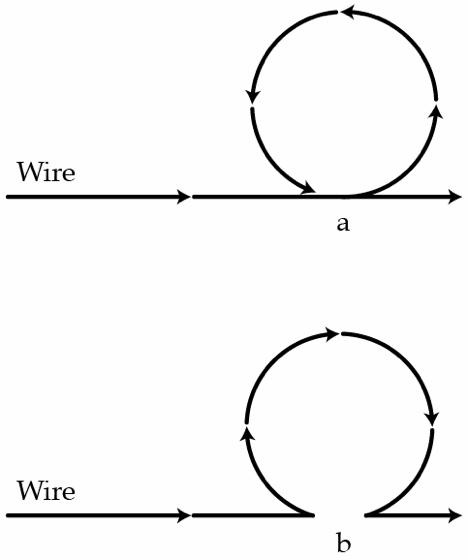A worker that maintains trolleys has to twist one part of the wiring in order to tighten up the wires. He knows that a direct current that goes through wires induces magnetic field and he wants to minimize the magnetic field in the loop to avoid any side effects.

The worker can twist the wire in two ways shown in the picture above. If the radius of the loop is $r$, and the magnetic field strength at a distance $r$ from a straight wire was $H_0 = 10~\mbox{A/m}$, what is the difference between the magnetic field strength in the middle of the loop in the case a) and b) in A/m?

Details and assumptions

• Your answer should be the absolute value of the difference between the fields.
×